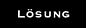# What is the value of 2 0

What is a solution with a hydrogen ion of 3.2 · 10 mol / L?One solution has a pH value of 7.0. What is the concentration of hydrogen ions in this solution?Practicing pH calculations:

1. c (NaOH) = 0.2 mol / L2. c (NH) = 0.01 mol / L3. c (acetic acid) = 0.5 mol / L4. c (formic acid) = 0.05 mol / L5. ENT6. c (HSO) = 0.05 mol / LCalculate the oxonium ion concentration and the pH value of an acetic acid solution with the concentration c = 0.25 mol / L.Does one have a big or a small one? How do or the pK value behave when diluting an acid?100 mL of a NaOH solution with unknown are mixed with a sulfuric acid solution (c = 1.0 mol / L), whereby 10 mL are consumed. What is the equivalent concentration of the given NaOH solution?Halfway to the a pH value = 5.4 was measured. Calculate the acid constant K. What is the pH of the solution if the concentration of the acid is c = 0.04 mol / L?Which titration curve results from the titration of a lactic acid solution?
(c = 0.01 mol / L) with a standard sodium hydroxide solution (c = 0.1 mol / L)?
The pH values ​​should be at 0; 0.1; 0.5; 0.9; 1.0 and 2.0
(i.e. with 10, 50, 90, 100 and 200% added standard solution).A solution of CO in water contains 1.2 · 10 mol CO in one liter of water. What is the pH of this solution?What is the pH value of a NaHCO solution with a concentration c (NaHCO) = 0.08 mol / L?:

1. What is the pH value of 1 liter of NaHPO solution (c = 0.5 mol / L)?2. What is the pH value after adding 0.1 mol of NaHPO to the solution from task a. a?